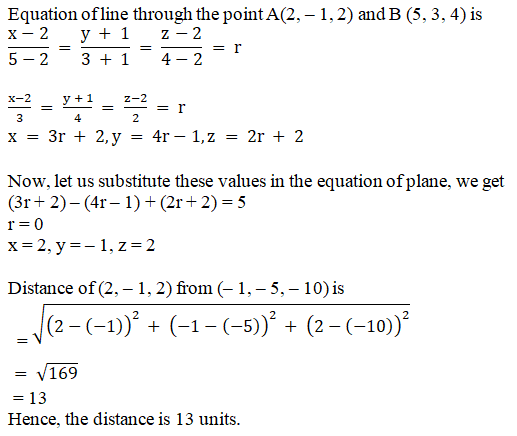# RD Sharma Solutions Class 12 The Plane Exercise 29.12

RD Sharma Solutions for Class 12 Maths Chapter 29 The Plane Exercise 29.12, is provided here for students to prepare and excel in their exams. Our solution module employs numerous shortcut tips and illustrations to explain all the exercise problems in the most understandable language. Students are advised to practice the RD Sharma Class 12 Solutions regularly to achieve their desired scores. Download the solutions pdf for free from the links given below.

## Download PDF of RD Sharma Solutions For Class 12 Maths Chapter 29 Exercise 12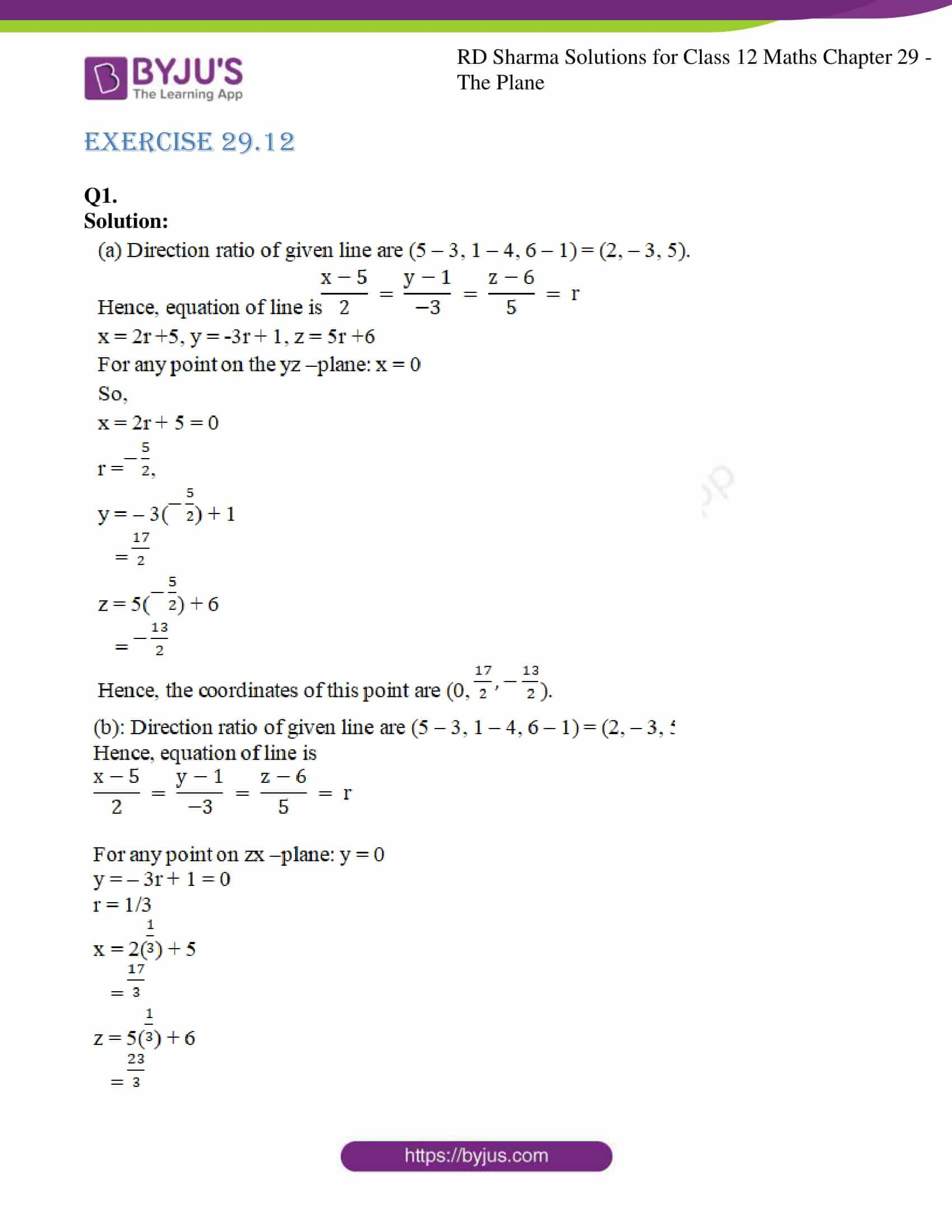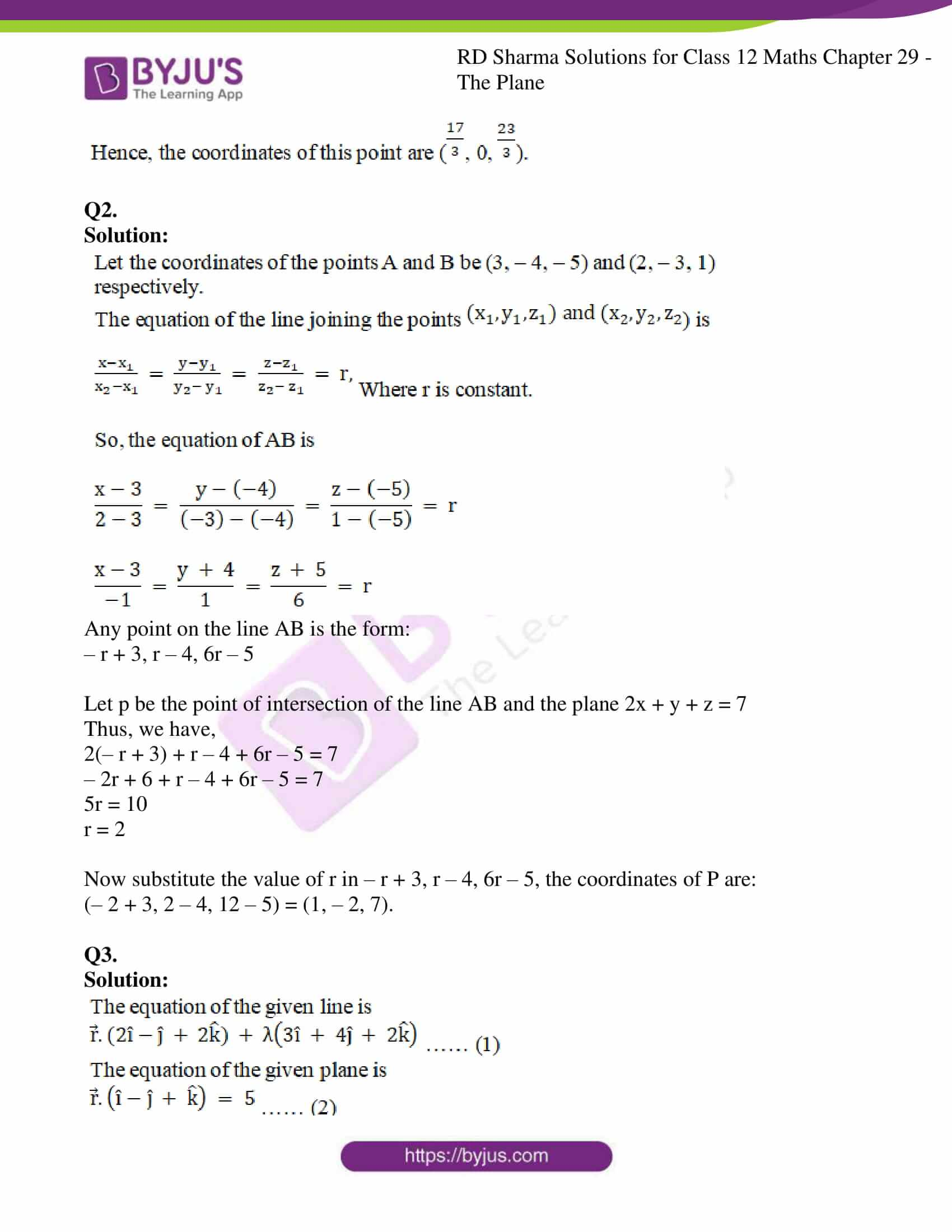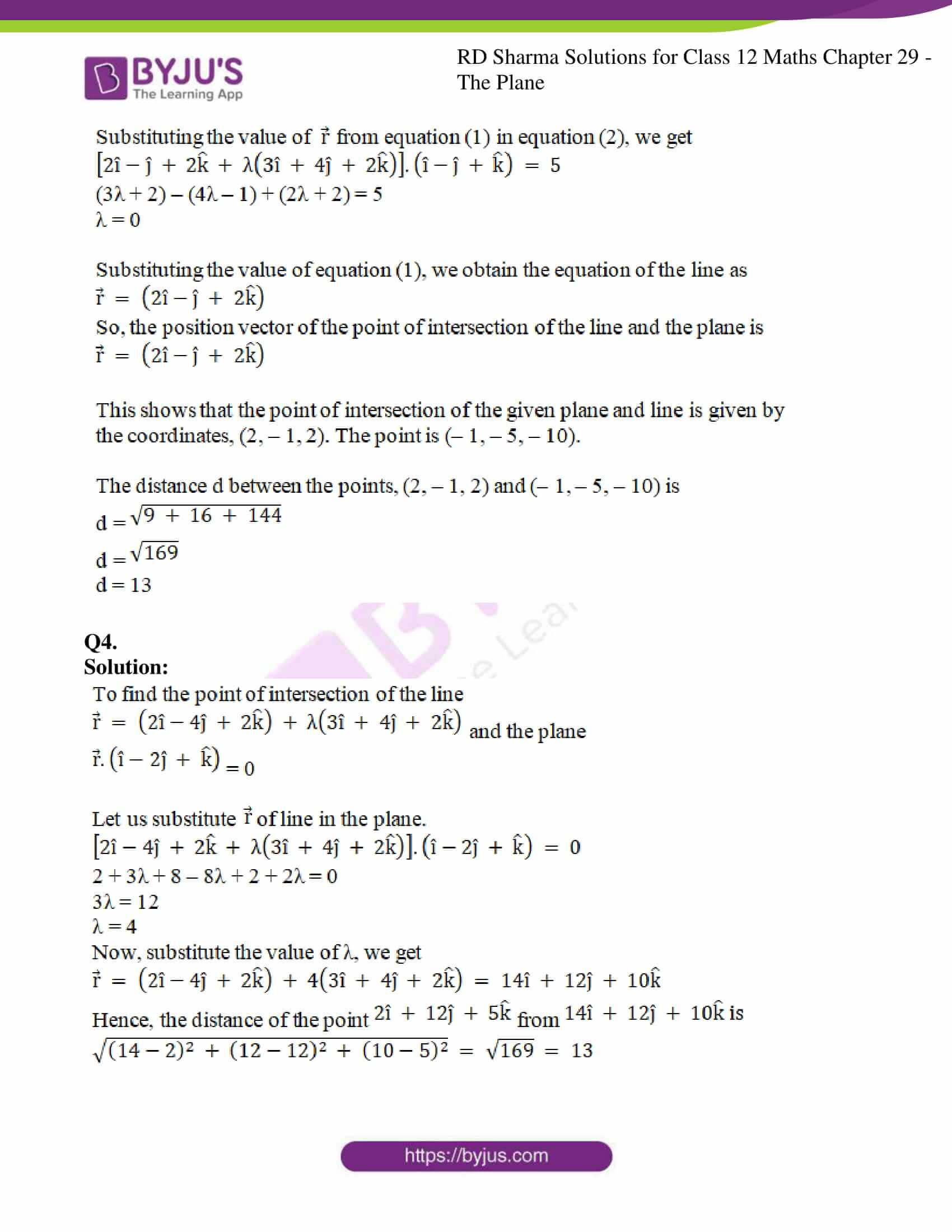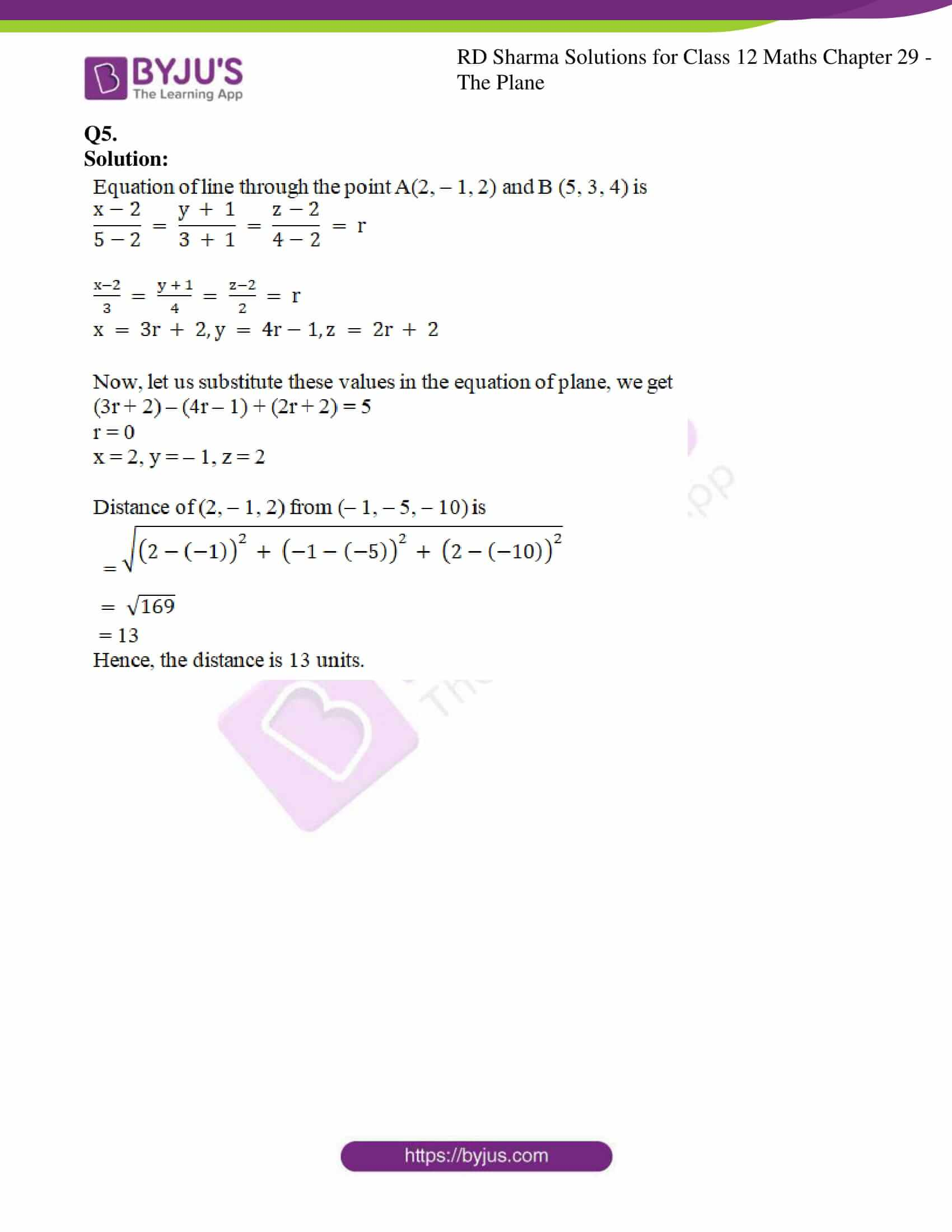### Access RD Sharma Solutions For Class 12 Maths Chapter 29 Exercise 12

EXERCISE 29.12

Q1.

Solution: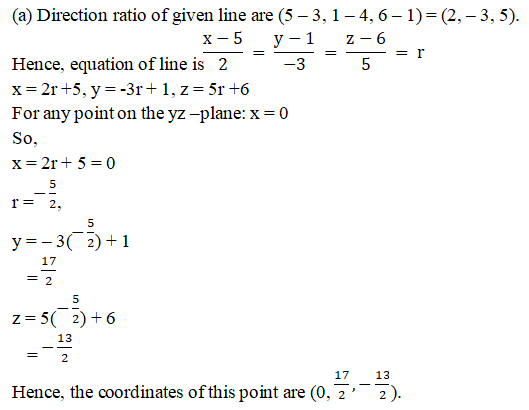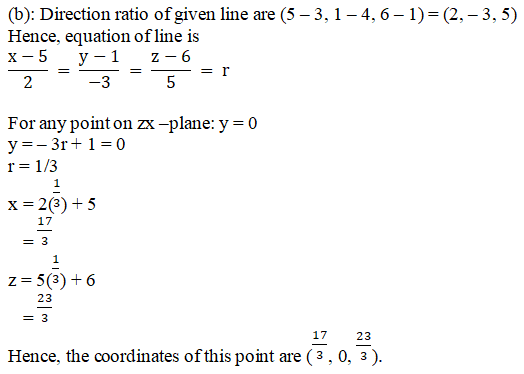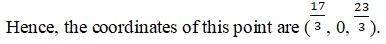Q2.

Solution: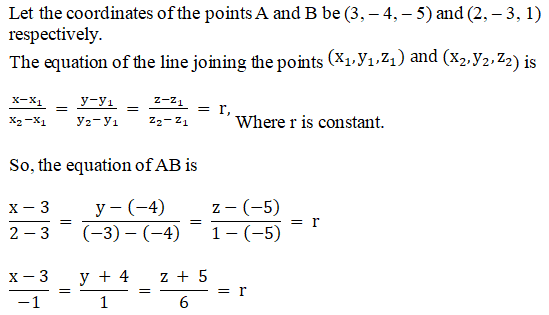Any point on the line AB is the form:

– r + 3, r – 4, 6r – 5

Let p be the point of intersection of the line AB and the plane 2x + y + z = 7

Thus, we have,

2(– r + 3) + r – 4 + 6r – 5 = 7

– 2r + 6 + r – 4 + 6r – 5 = 7

5r = 10

r = 2

Now substitute the value of r in – r + 3, r – 4, 6r – 5, the coordinates of P are:

(– 2 + 3, 2 – 4, 12 – 5) = (1, – 2, 7).

Q3.

Solution: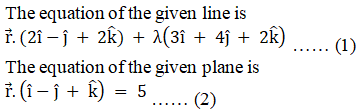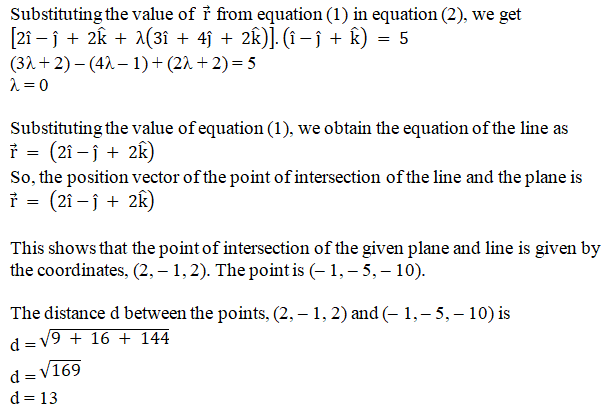Q4.

Solution: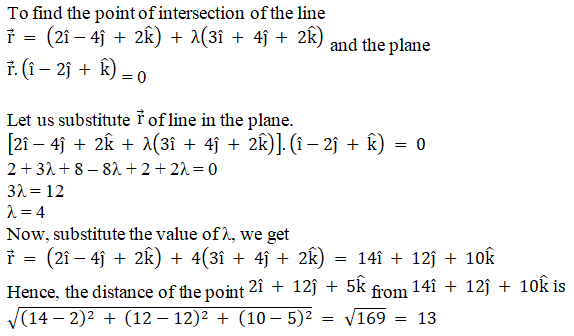Q5.

Solution: